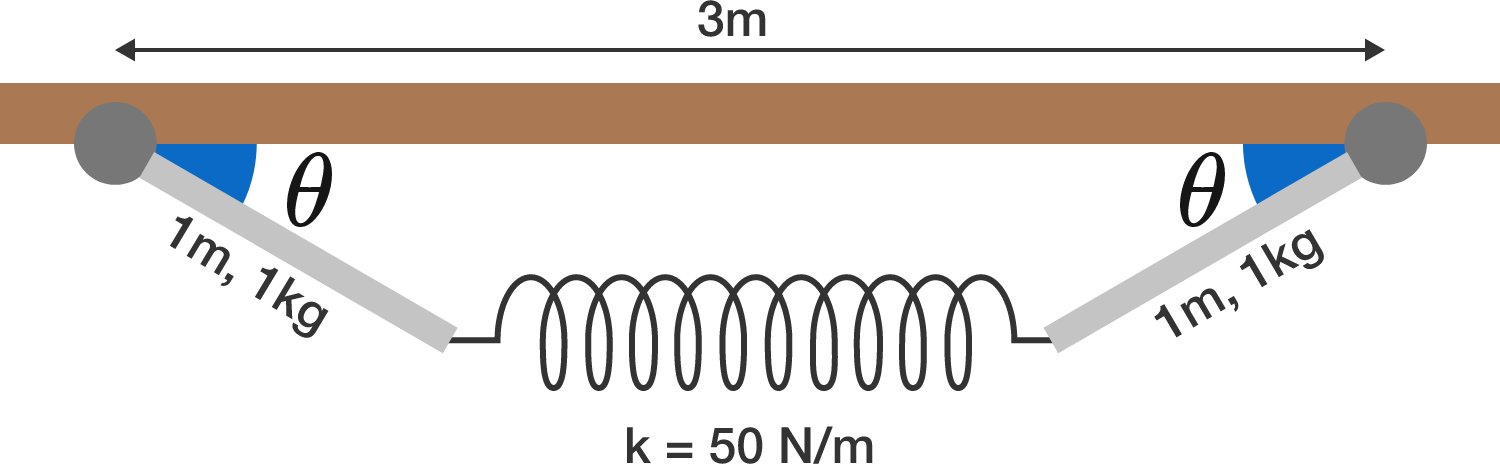# 2 Rods, 1 Spring

Two identical, massive rods are hinged at one end and connected together by a massless ideal spring.

If the system is in static equilibrium, what is the angle $\theta$ (in degrees)?


Details and Assumptions:

• Each rod is $1 \text{ m}$ long and has a mass of $1 \text{ kg}.$
• The spring constant is $k = 50 \text{ N/m}$, and the spring's unstretched length is $1 \text{ m}.$
• The two hinges are $3 \text{ m}$ apart horizontally.
• The strength of gravity is $10 \text{ m/s}^2$ in the downward, vertical direction.×

Problem Loading...

Note Loading...

Set Loading...